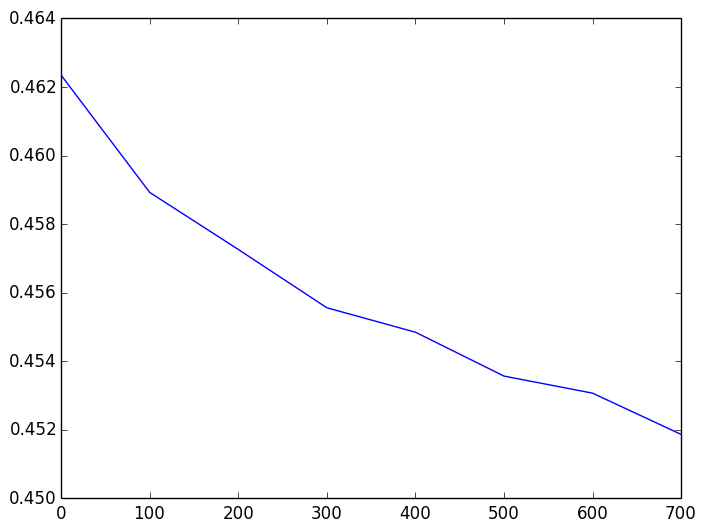The code below describes a general method by which a competitor in a machine learning competition can produce a highly misleading public leaderboard. I illustrate the problem through the Heritage Health Prize competition that was hosted by Kaggle and ran from April 2011 through April 2013.

Similar ideas apply to essentially any machine learning competition. The goal of this note is to illustrate the underlying problem. I made no effort to optimize the algorithm for this competition (which might lead to better results).

Details on how to avoid this problem and how to maintain an accurate leaderboard are the subject of a recent paper.

I don't have the actual data for the heritage health prize. So everything I'm going to say applies only under several assumptions on the actual data that seem reasonable to me. The general idea would've applied to the actual data as well, but the exact results would've been different.

In :
# score function
score(x,y) = sqrt(sum(log((1+x)./(1+y)).^2)/length(x))

# number of test samples
N = 70943
# used for the public leaderboard
n = int(0.3*N)

# score best constant predictor
const_benchmark = 0.486459
# best constant
const_value = 0.209179
# all zeros prediction
zeros_benchmark = 0.522226;


Based on these values, we can make some inferences about the holdout values $a_1,\dots,a_n.$ Specifically, the best constant benchmark c must satisfy (as we can see by taking derivatives) $$\log(1+c) = \frac 1n\sum_{i=1}^n \log(1+a_i)$$ Assuming that c=0.209179 as suggested by Kaggle, this pins down the mean (or first moment) of the $\log(1+a_i)$ values. Similarly, the zeros benchmark pins down the second moment $$0.522226 = \frac 1n\sum_{i=1}^n \log(1+a_i)^2$$

In :
first_moment = log(1+const_value)
second_moment = zeros_benchmark;


## A simple model for the holdout values¶

As we don't know the true holdout values, we instead sample them from a reasonable probabilistic model for the sake of this exercise.

For each $i=1,\dots,n$, we do the following independently:

• With probability $1-p$, we put $\log(1+a_i)=0,$
• with probability $p$, we sample $\log(1+a_i)$ from $\mathrm{Unif}([0,t])$.

This seems reasonable, because most patients don't go to the hospital at all (so we're in the first case). With probability $p$ the patient goes to the hospital in which case the log-duration might be roughly uniform in some interval. This doesn't account for very long hospital stays, but the score function treats all long enough stays roughly the same.

The first moment of the above distribution is equal to $pt/2$. The second moment is equal to $pt^2/3$. Given the above information, we can solve for $p$ and $t$. Using those parameters, we can sample holdout values.

In :
t = 3*second_moment/(2*first_moment)
p = 2*first_moment/t

# sample holdout values
solution = exp(t*rand(n) .* float(rand(n) .< p))-1;


## Boosting attack¶

The boosting attack takes two starting solutions $v_1$ and $v_2$ and tries to improve on the mean score achieved by those two solutions. It does so by trying out random combinations of the two vectors and selecting those that improve on the mean score. A final step aggregates all improving combinations into a single combination using a majority vote.

In :
# select coordinate from v1 if where v is 1 and from v2 where v is 0
combine(v,v1,v2) = v1 .* v + v2 .* (1-v)

function boost(v1,v2,k,score)
m = mean([score(v1),score(v2)])
A = rand(0:1,(length(v1),k))
# select columns of A that give better than mean score
a = filter(i -> score(combine(A[:,i],v1,v2)) < m,[1:k])
# take majority vote over all selected columns
v = float(A[:,a] * ones(length(a)) .> length(a)/2.0)
return combine(v,v1,v2)
end

Out:
boost (generic function with 1 method)
In :
# our score function
s(x) = round(score(solution,x),5)

Out:
s (generic function with 1 method)

We can see how this works below. We choose as the starting point two random perturbations of the true solution. Any two solutions will work instead provided that they are "sufficiently different". The boosting attack will approach the best "combination" of $v_1$ and $v_2.$ So, if the two solutions are too similar, the improvement will be small.

In :
vals = [1,100,200,300,400,500,600,700]
function expmt()
v1 = solution + 1.15 * rand(n)
v2 = solution + 1.15 * rand(n)
return Float64[ s(boost(v1,v2,i,s)) for i in vals ]
end

Out:
expmt (generic function with 1 method)
In :
reps = 10
S = zeros(reps,length(vals))
for i in 1:reps
S[i,:] = expmt()
end
means = [mean(S[:,j]) for j in 1:length(vals)]
stds = [std(S[:,j]) for j in 1:length(vals)];

In :
using PyPlot

INFO: Loading help data...

In :
plot(vals,means)Out:
1-element Array{Any,1}:
PyObject <matplotlib.lines.Line2D object at 0x119eaeb10>
In :
means

Out:
8-element Array{Any,1}:
0.462311
0.458926
0.457263
0.455562
0.454849
0.453569
0.453071
0.451868

### Getting to rank 1¶

This seems to require a lot more submissions and we can iterate the boosting attack.

In :
scores = Float64[]
for i in 1:reps
v1 = solution + 1.15 * rand(n)
v2 = solution + 1.15 * rand(n)
v21 = boost(v1,v2,500,s)
v22 = boost(v1,v2,500,s)
v31 = boost(v21,v22,500,s)
v32 = boost(v21,v22,500,s)
v4 = boost(v31,v32,500,s)
push!(scores,s(v4))
end
mean(scores)

Out:
0.4425490000000001
In [ ]: